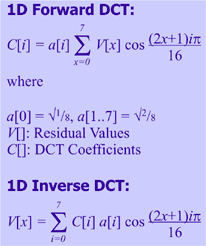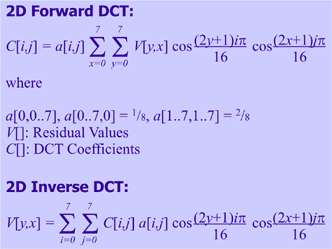# AIC - Discrete Cosine Transform and Quantisation

The Advanced Image Coding codec uses the Discrete Cosine Transform (DCT) to transform a 8x8 residual block into a set of coefficients to cosine functions with increasing frequencies. Below you will find a short introduction to the DCT. For more detailed information there are plenty of resources available on the internet; see the Resources and links section.

## 1-Dimensional DCT

The 1-dimensional Forward DCT transforms a row of 8 residual values (V) into a row of 8 coefficients(C):The following figure shows the result of the FDCT, applied to a sample row. The residual values are represented using shades of gray (for clarity, only positive values are used here)

 Sample rowResidual values 121 58 61 113 171 200 226 246 DCT coefficients 61 -175 43 49 37 10 5 5

The resulting DCT coefficients don't look better compressible at all. The clue to compression, however, can be seen in the decreasing magnitude of the coefficients. The later coefficients are much smaller than the first ones, which might indicate that these do not contribute much to the image quality. The following graphs clarify this:The red dots represent the original residual values, also shown at the bottom of each graph. The blue line represent the reconstructed residual values after performing an Inverse DCT on several DCT coefficients. In the first graph, only the first DCT coefficient (the DC coefficients) is used to reconstruct the values. In each following graph, an additional coefficient (AC coefficient) is added to the reconstruction. The first graph shows a straight line through the average residual values, which means that the DC coefficient represents the average of an entire row of values. By adding AC coefficients, detail is added to the image and the blue line moves closer to the original red dots. By using only 4 of the 8 coefficients, the reconstructed line is already close to the original. From the fifth graph onward, the subsequent improvements become smaller and less noticeable.
This is the key to compression. Since the higher-order (high-frequency) DCT coefficients contribute less information to the image, they can be discarded while still producing a close approximation of the original.

## Quantisation

But completely discarding coefficients is not always desirable. In certain types of images with high contrast, like textual images or cartoons, the high frequency coefficients are important to the image detail and cannot be discarded. This is why JPEG, and AIC, use quantisation, which is just a fancy word for dividing in this context. Each coefficient gets divided by a certain value. The higher this value, the smaller the results will be. This will make the coefficients better compressible, but also reduces image quality because the coefficients cannot be reconstructed faithfully. When you choose a quality level in JPEG or AIC, you actually set the amount of quantisation used.

JPEG uses the DCT to transform pixel values instead of residual values. It also uses a non-uniform quantisation method by which high frequency coefficients (the later coefficients) are quantised with higher values than low frequency ones.

AIC performs the DCT on residual values. Several tests have shown that uniform quantisation is more appropriate in this case. In AIC, all coefficients are quantised by the same value.

## 2-Dimensional DCT

The 1-dimensional DCT discussed above only takes advantage of correlation between residual values in a row. Better compression can be achieved when we take both the horizontal and vertical correlation between residual values into account. This is done by performing a 2-dimensional DCT on a block of 8x8 residual values:However, a 2D DCT can also be implemented by first applying a 1D DCT on all rows, followed by a 1D DCT on all columns from the result of the first step. This is much faster than implementing a 2D DCT directly.

The DCT code used in the AIC codec is based on the code in the JPEG reference software from the Independent JPEG Group. This reference software supports different algorithms. AIC only uses the floating point algorithm since it produces the highest quality images. It's a bit slower than the other algorithms, but on modern computers, floating point calculations are performed much faster than in the old days. To speed up the calculations, the AAN (Arai, Agui and Nakajima) algorithm is used to calculate the DCT.

Finally, in the last step, the DCT coefficients and prediction modes are encoded to the stream using Context Adaptive Binary Arithmetic Coding(CABAC). In JPEG the DCT coefficients are transmitted in zigzag order to form runs of zeros which can be encoded using run length encoding. The CABAC codec does not use run length encoding, so there is no need to reorder the DCT coefficients. So in AIC, the coefficients are transmitted in scan line order.